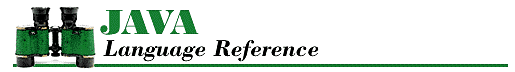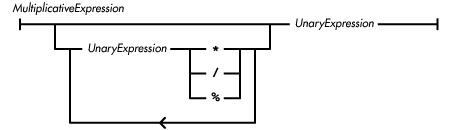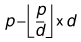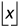home | O'Reilly's CD bookshelfs | FreeBSD | Linux | Cisco | Cisco Exam

### 4.5 Multiplicative Operators

The multiplicative operators in Java are binary operators that are used for multiplication (*), division (/), and the remainder operation (%). The multiplicative operators appear in multiplicative expressions:The multiplicative operators are equal in precedence and are evaluated from left to right.

References Unary Operators; Order of Operations

### Multiplication Operator *

The binary multiplication operator * produces a pure value that is the product of its operands. The * operator may appear in a multiplicative expression. The multiplication operator never throws an exception.

Here is an example that uses the multiplication operator:

```int doubleIt(int x) {
return x*2;
}
```

The types of both operands of the multiplication operator must be arithmetic types, or a compile-time error occurs. The * operator may perform type conversions on its operands:

• If either operand is of type double, the other operand is converted to double and the operation produces a double value.

• Otherwise, if either operand is of type float, the other operand is converted to float and the operation produces a float value.

• Otherwise, if either operand is of type long, the other operand is converted to long and the operation produces a long value.

• Otherwise, both operands are converted to int and the operation produces an int value.

If the multiplication of integer data overflows, the low order bits of the product are returned; no exception is thrown. The most significant bit of the low order bits is treated as a sign bit. When overflow occurs, the sign of the number produced may not be the same as the sign of the mathematically correct product, due to the limitations of the two's complement representation used for integer data.

The multiplication of floating-point data is governed by the following rules:

• If either operand is not-a-number (NaN), the product is NaN.

• If neither operand is NaN and if both operands have the same sign, the product is positive.

• If neither operand is NaN and if the operands have different signs, the product is negative.

• If one of the operands is positive or negative infinity and the other operand is positive or negative zero, the product is NaN.

• If one of the operands is an infinity value and the other operand is neither zero nor NaN, the product is either positive or negative infinity, as determined by the rules governing the sign of products.

• If neither operand is a zero value, an infinity value, or NaN, the product is rounded to the nearest representable value. If the magnitude of the product is too large to be represented, the operation overflows and an infinity value of the appropriate sign is produced. If the magnitude of the product is too small to be represented, the operation underflows and a zero value of the appropriate sign is produced.

References Arithmetic Types

### Division Operator /

The binary division operator / produces a pure value that is the quotient of its operands. The left operand is the dividend and the right operand is the divisor. The / operator may appear in a multiplicative expression.

Here is an example that uses the division operator:

```int halfIt(int x) {
return x/2;
}
```

The types of both operands of the division operator must be arithmetic types, or a compile-time error occurs. The / operator may perform type conversions on its operands:

• If either operand is of type double, the other operand is converted to double and the operation produces a double value.

• Otherwise, if either operand is of type float, the other operand is converted to float and the operation produces a float value.

• Otherwise, if either operand is of type long, the other operand is converted to long and the operation produces a long value.

• Otherwise, both operands are converted to int and the operation produces an int value.

The division of integer data rounds toward zero. If the divisor of an integer division operator is zero, an ArithmeticException is thrown. If the dividend is Integer.MIN_VALUE or Long.MIN_VALUE and the divisor is -1, the quotient produced is Integer.MIN_VALUE or Long.MIN_VALUE, due to the limitations of the two's complement representation used for integer data.

The division of floating-point data is governed by the following rules:

• If either operand is not-a-number (NaN), the quotient is NaN.

• If neither operand is NaN and if both operands have the same sign, the quotient is positive.

• If neither operand is NaN and if the operands have different signs, the quotient is negative.

• If both of the operands are positive or negative infinity, the quotient is NaN.

• If the dividend is an infinity value and the divisor is a finite number, the quotient is either positive or negative infinity, as determined by the rules governing the sign of quotients.

• If the dividend is a finite number and the divisor is an infinity value, the quotient is either positive or negative zero, as determined by the rules governing the sign of quotients.

• If the divisor is positive or negative zero and the dividend is not zero or NaN, the quotient is either positive or negative infinity, as determined by the rules governing the sign of quotients.

• If both operands are zero values, the quotient is NaN.

• If the dividend is a zero value and the divisor is a non-zero finite number, the quotient is either positive or negative zero, as determined by the rules governing the sign of quotients.

• If the dividend is a non-zero finite number and the divisor is a zero value, the quotient is either positive or negative infinity, as determined by the rules governing the sign of quotients.

• If neither operand is a zero value, an infinity value, or NaN, the quotient is rounded to the nearest representable value. If the magnitude of the quotient is too large to be represented, the operation overflows and an infinity value of the appropriate sign is produced. If the magnitude of the quotient is too small to be represented, the operation underflows and a zero value of the appropriate sign is produced.

References Arithmetic Types; Integer; Long; Runtime exceptions

### Remainder Operator %

The binary remainder operator % produces a pure value that is the remainder from an implied division of its operands. The left operand is the dividend and the right operand is the divisor. The % operator may appear in a multiplicative expression.

Here is an example that uses the remainder operator:

```// format seconds into hours, minutes and seconds
String formatTime(int t) {
int minutes, seconds;
seconds = t%60;
t /= 60;
minutes = t%60;
return t/60 + ":" + minutes + ":" + seconds;
}
```

The types of both operands of the remainder operator must be arithmetic types, or a compile-time error occurs. The % operator may perform type conversions on its operands:

• If either operand is of type double, the other operand is converted to double and the operation produces a double value.

• Otherwise, if either operand is of type float, the other operand is converted to float and the operation produces a float value.

• Otherwise, if either operand is of type long, the other operand is converted to long and the operation produces a long value.

• Otherwise, both operands are converted to int and the operation produces an int value.

When the remainder operation is performed on integer data, the following expression is guaranteed to produce the same value as a%b:

```a-((a/b)*b)
```

The sign of the value produced by the remainder operator is always the sign of the dividend. The magnitude of the value produced by the remainder operator is always less than the absolute value of the divisor. If the divisor is zero, an ArithmeticException is thrown.

Unlike C/C++, Java provides a remainder operation for floating-point data. The remainder of floating-point data is computed in a manner similar to the remainder of integer data. The remainder operation uses a truncating division to compute its value. This is unlike the IEEE 754 remainder operation, which uses a rounding division. The IEEE remainder operation is provided by the Math.IEEEremainder() method.

The computation of the remainder of double and float data is governed by the following rules:

• If either operand is not-a-number (NaN), the remainder is NaN.

• If neither operand is NaN, the sign of the remainder is the same as the sign of the dividend.

• If the dividend is positive or negative infinity or the divisor is positive or negative zero, the remainder is NaN.

• If the dividend is a finite number and the divisor is an infinity value, the remainder is equal to the dividend.

• If the dividend is a zero value and the divisor is a finite number, the remainder is equal to the dividend.

• If neither operand is a zero value, an infinity value, or NaN, the remainder is computed according to the following mathematical formula:p is the dividend and d is the divisor. The notationmeans the greatest integer less than or equal to x ; this is called the floor operation.

References Arithmetic Types; Math; Runtime exceptions